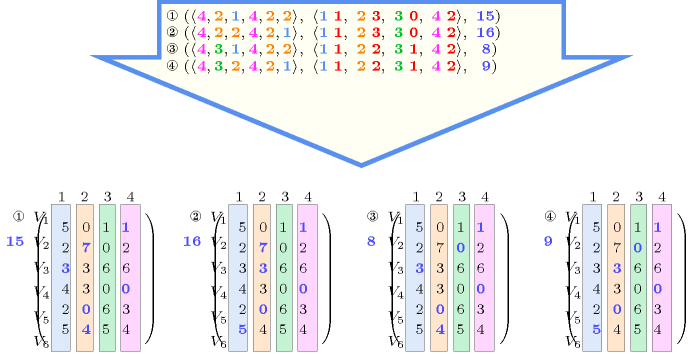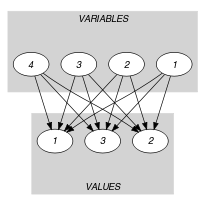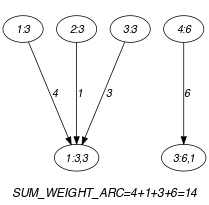## 5.167. global_cardinality_with_costs

Origin
Constraint

$\mathrm{𝚐𝚕𝚘𝚋𝚊𝚕}_\mathrm{𝚌𝚊𝚛𝚍𝚒𝚗𝚊𝚕𝚒𝚝𝚢}_\mathrm{𝚠𝚒𝚝𝚑}_\mathrm{𝚌𝚘𝚜𝚝𝚜}\left(\mathrm{𝚅𝙰𝚁𝙸𝙰𝙱𝙻𝙴𝚂},\mathrm{𝚅𝙰𝙻𝚄𝙴𝚂},\mathrm{𝙼𝙰𝚃𝚁𝙸𝚇},\mathrm{𝙲𝙾𝚂𝚃}\right)$

Synonyms

$\mathrm{𝚐𝚌𝚌𝚌}$, $\mathrm{𝚌𝚘𝚜𝚝}_\mathrm{𝚐𝚌𝚌}$.

Arguments
 $\mathrm{𝚅𝙰𝚁𝙸𝙰𝙱𝙻𝙴𝚂}$ $\mathrm{𝚌𝚘𝚕𝚕𝚎𝚌𝚝𝚒𝚘𝚗}\left(\mathrm{𝚟𝚊𝚛}-\mathrm{𝚍𝚟𝚊𝚛}\right)$ $\mathrm{𝚅𝙰𝙻𝚄𝙴𝚂}$ $\mathrm{𝚌𝚘𝚕𝚕𝚎𝚌𝚝𝚒𝚘𝚗}\left(\mathrm{𝚟𝚊𝚕}-\mathrm{𝚒𝚗𝚝},\mathrm{𝚗𝚘𝚌𝚌𝚞𝚛𝚛𝚎𝚗𝚌𝚎}-\mathrm{𝚍𝚟𝚊𝚛}\right)$ $\mathrm{𝙼𝙰𝚃𝚁𝙸𝚇}$ $\mathrm{𝚌𝚘𝚕𝚕𝚎𝚌𝚝𝚒𝚘𝚗}\left(𝚒-\mathrm{𝚒𝚗𝚝},𝚓-\mathrm{𝚒𝚗𝚝},𝚌-\mathrm{𝚒𝚗𝚝}\right)$ $\mathrm{𝙲𝙾𝚂𝚃}$ $\mathrm{𝚍𝚟𝚊𝚛}$
Restrictions
 $\mathrm{𝚛𝚎𝚚𝚞𝚒𝚛𝚎𝚍}$$\left(\mathrm{𝚅𝙰𝚁𝙸𝙰𝙱𝙻𝙴𝚂},\mathrm{𝚟𝚊𝚛}\right)$ $|\mathrm{𝚅𝙰𝙻𝚄𝙴𝚂}|>0$ $\mathrm{𝚛𝚎𝚚𝚞𝚒𝚛𝚎𝚍}$$\left(\mathrm{𝚅𝙰𝙻𝚄𝙴𝚂},\left[\mathrm{𝚟𝚊𝚕},\mathrm{𝚗𝚘𝚌𝚌𝚞𝚛𝚛𝚎𝚗𝚌𝚎}\right]\right)$ $\mathrm{𝚍𝚒𝚜𝚝𝚒𝚗𝚌𝚝}$$\left(\mathrm{𝚅𝙰𝙻𝚄𝙴𝚂},\mathrm{𝚟𝚊𝚕}\right)$ $\mathrm{𝚅𝙰𝙻𝚄𝙴𝚂}.\mathrm{𝚗𝚘𝚌𝚌𝚞𝚛𝚛𝚎𝚗𝚌𝚎}\ge 0$ $\mathrm{𝚅𝙰𝙻𝚄𝙴𝚂}.\mathrm{𝚗𝚘𝚌𝚌𝚞𝚛𝚛𝚎𝚗𝚌𝚎}\le |\mathrm{𝚅𝙰𝚁𝙸𝙰𝙱𝙻𝙴𝚂}|$ $\mathrm{𝚛𝚎𝚚𝚞𝚒𝚛𝚎𝚍}$$\left(\mathrm{𝙼𝙰𝚃𝚁𝙸𝚇},\left[𝚒,𝚓,𝚌\right]\right)$ $\mathrm{𝚒𝚗𝚌𝚛𝚎𝚊𝚜𝚒𝚗𝚐}_\mathrm{𝚜𝚎𝚚}$$\left(\mathrm{𝙼𝙰𝚃𝚁𝙸𝚇},\left[𝚒,𝚓\right]\right)$ $\mathrm{𝙼𝙰𝚃𝚁𝙸𝚇}.𝚒\ge 1$ $\mathrm{𝙼𝙰𝚃𝚁𝙸𝚇}.𝚒\le |\mathrm{𝚅𝙰𝚁𝙸𝙰𝙱𝙻𝙴𝚂}|$ $\mathrm{𝙼𝙰𝚃𝚁𝙸𝚇}.𝚓\ge 1$ $\mathrm{𝙼𝙰𝚃𝚁𝙸𝚇}.𝚓\le |\mathrm{𝚅𝙰𝙻𝚄𝙴𝚂}|$ $|\mathrm{𝙼𝙰𝚃𝚁𝙸𝚇}|=|\mathrm{𝚅𝙰𝚁𝙸𝙰𝙱𝙻𝙴𝚂}|*|\mathrm{𝚅𝙰𝙻𝚄𝙴𝚂}|$
Purpose

Each value $\mathrm{𝚅𝙰𝙻𝚄𝙴𝚂}\left[i\right].\mathrm{𝚟𝚊𝚕}$ should be taken by exactly $\mathrm{𝚅𝙰𝙻𝚄𝙴𝚂}\left[i\right].\mathrm{𝚗𝚘𝚌𝚌𝚞𝚛𝚛𝚎𝚗𝚌𝚎}$ variables of the $\mathrm{𝚅𝙰𝚁𝙸𝙰𝙱𝙻𝙴𝚂}$ collection. In addition the $\mathrm{𝙲𝙾𝚂𝚃}$ of an assignment is equal to the sum of the elementary costs associated with the fact that we assign variable $i$ of the $\mathrm{𝚅𝙰𝚁𝙸𝙰𝙱𝙻𝙴𝚂}$ collection to the ${j}^{th}$ value of the $\mathrm{𝚅𝙰𝙻𝚄𝙴𝚂}$ collection. These elementary costs are given by the $\mathrm{𝙼𝙰𝚃𝚁𝙸𝚇}$ collection.

Example
$\left(\begin{array}{c}〈3,3,3,6〉,\hfill \\ 〈\begin{array}{cc}\mathrm{𝚟𝚊𝚕}-3\hfill & \mathrm{𝚗𝚘𝚌𝚌𝚞𝚛𝚛𝚎𝚗𝚌𝚎}-3,\hfill \\ \mathrm{𝚟𝚊𝚕}-5\hfill & \mathrm{𝚗𝚘𝚌𝚌𝚞𝚛𝚛𝚎𝚗𝚌𝚎}-0,\hfill \\ \mathrm{𝚟𝚊𝚕}-6\hfill & \mathrm{𝚗𝚘𝚌𝚌𝚞𝚛𝚛𝚎𝚗𝚌𝚎}-1\hfill \end{array}〉,\hfill \\ 〈\begin{array}{ccc}𝚒-1\hfill & 𝚓-1\hfill & 𝚌-4,\hfill \\ 𝚒-1\hfill & 𝚓-2\hfill & 𝚌-1,\hfill \\ 𝚒-1\hfill & 𝚓-3\hfill & 𝚌-7,\hfill \\ 𝚒-2\hfill & 𝚓-1\hfill & 𝚌-1,\hfill \\ 𝚒-2\hfill & 𝚓-2\hfill & 𝚌-0,\hfill \\ 𝚒-2\hfill & 𝚓-3\hfill & 𝚌-8,\hfill \\ 𝚒-3\hfill & 𝚓-1\hfill & 𝚌-3,\hfill \\ 𝚒-3\hfill & 𝚓-2\hfill & 𝚌-2,\hfill \\ 𝚒-3\hfill & 𝚓-3\hfill & 𝚌-1,\hfill \\ 𝚒-4\hfill & 𝚓-1\hfill & 𝚌-0,\hfill \\ 𝚒-4\hfill & 𝚓-2\hfill & 𝚌-0,\hfill \\ 𝚒-4\hfill & 𝚓-3\hfill & 𝚌-6\hfill \end{array}〉,14\hfill \end{array}\right)$

The $\mathrm{𝚐𝚕𝚘𝚋𝚊𝚕}_\mathrm{𝚌𝚊𝚛𝚍𝚒𝚗𝚊𝚕𝚒𝚝𝚢}_\mathrm{𝚠𝚒𝚝𝚑}_\mathrm{𝚌𝚘𝚜𝚝𝚜}$ constraint holds since:

• Values 3, 5 and 6 respectively occur 3, 0 and 1 times within the collection $〈3,3,3,6〉$.

• The $\mathrm{𝙲𝙾𝚂𝚃}$ argument corresponds to the sum of the costs respectively associated with the first, second, third and fourth items of $〈3,3,3,6〉$, namely 4, 1, 3 and 6.

All solutions

Figure 5.167.1 gives all solutions to the following non ground instance of the $\mathrm{𝚐𝚕𝚘𝚋𝚊𝚕}_\mathrm{𝚌𝚊𝚛𝚍𝚒𝚗𝚊𝚕𝚒𝚝𝚢}_\mathrm{𝚠𝚒𝚝𝚑}_\mathrm{𝚌𝚘𝚜𝚝𝚜}$ constraint:

${V}_{1}\in \left[3,4\right]$, ${V}_{2}\in \left[2,3\right]$, ${V}_{3}\in \left[1,2\right]$, ${V}_{4}\in \left[2,4\right]$, ${V}_{5}\in \left[2,3\right]$, ${V}_{6}\in \left[1,2\right]$,

${O}_{1}\in \left[1,1\right]$, ${O}_{2}\in \left[2,3\right]$, ${O}_{3}\in \left[0,1\right]$, ${O}_{4}\in \left[2,3\right]$,

$C\in \left[0,16\right]$,

$\mathrm{𝚐𝚕𝚘𝚋𝚊𝚕}_\mathrm{𝚌𝚊𝚛𝚍𝚒𝚗𝚊𝚕𝚒𝚝𝚢}_\mathrm{𝚠𝚒𝚝𝚑}_\mathrm{𝚌𝚘𝚜𝚝𝚜}$$\left(〈{V}_{1},{V}_{2},{V}_{3},{V}_{4},{V}_{5},{V}_{6}〉$,

$〈1{O}_{1},2{O}_{2},3{O}_{3},4{O}_{4}〉$,

$〈115,120,131,141$

$212,227,230,242$

$313,323,336,346$

$414,423,430,440$

$512,520,536,543$

$615,624,635,644〉,C\right)$.

##### Figure 5.167.1. All solutions corresponding to the non ground example of the $\mathrm{𝚐𝚕𝚘𝚋𝚊𝚕}_\mathrm{𝚌𝚊𝚛𝚍𝚒𝚗𝚊𝚕𝚒𝚝𝚢}_\mathrm{𝚠𝚒𝚝𝚑}_\mathrm{𝚌𝚘𝚜𝚝𝚜}$ constraint of the All solutions slotTypical
 $|\mathrm{𝚅𝙰𝚁𝙸𝙰𝙱𝙻𝙴𝚂}|>1$ $\mathrm{𝚛𝚊𝚗𝚐𝚎}$$\left(\mathrm{𝚅𝙰𝚁𝙸𝙰𝙱𝙻𝙴𝚂}.\mathrm{𝚟𝚊𝚛}\right)>1$ $|\mathrm{𝚅𝙰𝙻𝚄𝙴𝚂}|>1$ $\mathrm{𝚛𝚊𝚗𝚐𝚎}$$\left(\mathrm{𝚅𝙰𝙻𝚄𝙴𝚂}.\mathrm{𝚗𝚘𝚌𝚌𝚞𝚛𝚛𝚎𝚗𝚌𝚎}\right)>1$ $\mathrm{𝚛𝚊𝚗𝚐𝚎}$$\left(\mathrm{𝙼𝙰𝚃𝚁𝙸𝚇}.𝚌\right)>1$ $|\mathrm{𝚅𝙰𝚁𝙸𝙰𝙱𝙻𝙴𝚂}|>|\mathrm{𝚅𝙰𝙻𝚄𝙴𝚂}|$
Arg. properties
• Functional dependency: $\mathrm{𝚅𝙰𝙻𝚄𝙴𝚂}.\mathrm{𝚗𝚘𝚌𝚌𝚞𝚛𝚛𝚎𝚗𝚌𝚎}$ determined by $\mathrm{𝚅𝙰𝚁𝙸𝙰𝙱𝙻𝙴𝚂}$.

• Functional dependency: $\mathrm{𝙲𝙾𝚂𝚃}$ determined by $\mathrm{𝚅𝙰𝚁𝙸𝙰𝙱𝙻𝙴𝚂}$, $\mathrm{𝚅𝙰𝙻𝚄𝙴𝚂}$ and $\mathrm{𝙼𝙰𝚃𝚁𝙸𝚇}$.

Usage

A classical utilisation of the $\mathrm{𝚐𝚕𝚘𝚋𝚊𝚕}_\mathrm{𝚌𝚊𝚛𝚍𝚒𝚗𝚊𝚕𝚒𝚝𝚢}_\mathrm{𝚠𝚒𝚝𝚑}_\mathrm{𝚌𝚘𝚜𝚝𝚜}$ constraint corresponds to the following assignment problem. We have a set of persons $𝒫$ as well as a set of jobs $𝒥$ to perform. Each job requires a number of persons restricted to a specified interval. In addition each person $p$ has to be assigned to one specific job taken from a subset ${𝒥}_{p}$ of $𝒥$. There is a cost ${C}_{pj}$ associated with the fact that person $p$ is assigned to job $j$. The previous problem is modelled with a single $\mathrm{𝚐𝚕𝚘𝚋𝚊𝚕}_\mathrm{𝚌𝚊𝚛𝚍𝚒𝚗𝚊𝚕𝚒𝚝𝚢}_\mathrm{𝚠𝚒𝚝𝚑}_\mathrm{𝚌𝚘𝚜𝚝𝚜}$ constraint where the persons and the jobs respectively correspond to the items of the $\mathrm{𝚅𝙰𝚁𝙸𝙰𝙱𝙻𝙴𝚂}$ and $\mathrm{𝚅𝙰𝙻𝚄𝙴𝚂}$ collection.

The $\mathrm{𝚐𝚕𝚘𝚋𝚊𝚕}_\mathrm{𝚌𝚊𝚛𝚍𝚒𝚗𝚊𝚕𝚒𝚝𝚢}_\mathrm{𝚠𝚒𝚝𝚑}_\mathrm{𝚌𝚘𝚜𝚝𝚜}$ constraint can also be used for modelling a conjunction $\mathrm{𝚊𝚕𝚕𝚍𝚒𝚏𝚏𝚎𝚛𝚎𝚗𝚝}$$\left({𝚇}_{\mathtt{1}},{𝚇}_{\mathtt{2}},\cdots ,{𝚇}_{𝚗}\right)$ and ${\alpha }_{1}·{𝚇}_{\mathtt{1}}+{\alpha }_{2}·{𝚇}_{\mathtt{2}}+\cdots +{\alpha }_{n}·{𝚇}_{𝚗}=\mathrm{𝙲𝙾𝚂𝚃}$. For this purpose we set the domain of the $\mathrm{𝚗𝚘𝚌𝚌𝚞𝚛𝚛𝚎𝚗𝚌𝚎}$ variables to $\left\{0,1\right\}$ and the cost attribute $𝚌$ of a variable ${𝚇}_{𝚒}$ and one of its potential value $𝚓$ to ${\alpha }_{i}·𝚓$. In practice this can be used for the magic squares and the magic hexagon problems where all the ${\alpha }_{i}$ are set to 1.

Algorithm

A filtering algorithm achieving arc-consistency independently on each side (i.e., the greater than or equal to side and the less than or equal to side) of the $\mathrm{𝚐𝚕𝚘𝚋𝚊𝚕}_\mathrm{𝚌𝚊𝚛𝚍𝚒𝚗𝚊𝚕𝚒𝚝𝚢}_\mathrm{𝚠𝚒𝚝𝚑}_\mathrm{𝚌𝚘𝚜𝚝𝚜}$ constraint is described in [Regin99a], [Regin02]. This algorithm assumes for each value a fixed minimum and maximum number of occurrences. If we rather have occurrence variables, the Reformulation slot explains how to also obtain some propagation from the cost variable back to the occurrence variables.

Reformulation

Let $n$ and $m$ respectively denote the number of items of the $\mathrm{𝚅𝙰𝚁𝙸𝙰𝙱𝙻𝙴𝚂}$ and of the $\mathrm{𝚅𝙰𝙻𝚄𝙴𝚂}$ collections. Let ${v}_{1},{v}_{2},\cdots ,{v}_{m}$ denote the values $\mathrm{𝚅𝙰𝙻𝚄𝙴𝚂}\left[1\right].\mathrm{𝚟𝚊𝚕},\mathrm{𝚅𝙰𝙻𝚄𝙴𝚂}\left[2\right].\mathrm{𝚟𝚊𝚕},\cdots ,\mathrm{𝚅𝙰𝙻𝚄𝙴𝚂}\left[m\right].\mathrm{𝚟𝚊𝚕}$. In addition let ${\mathrm{𝐿𝐼𝑁𝐸}}_{i}$ (with $i\in \left[1,n\right]$) denote the values $〈\mathrm{𝙼𝙰𝚃𝚁𝙸𝚇}\left[m·\left(i-1\right)+1\right].𝚌,\mathrm{𝙼𝙰𝚃𝚁𝙸𝚇}\left[m·\left(i-1\right)+2\right].𝚌,\cdots ,\mathrm{𝙼𝙰𝚃𝚁𝙸𝚇}\left[m·i\right].𝚌〉$, i.e., line $i$ of the matrix $\mathrm{𝙼𝙰𝚃𝚁𝙸𝚇}$.

By introducing $2·n$ auxiliary variables ${U}_{1},{U}_{2},\cdots ,{U}_{n}$ and ${C}_{1},{C}_{2},\cdots ,{C}_{n}$, the $\mathrm{𝚐𝚕𝚘𝚋𝚊𝚕}_\mathrm{𝚌𝚊𝚛𝚍𝚒𝚗𝚊𝚕𝚒𝚝𝚢}_\mathrm{𝚠𝚒𝚝𝚑}_\mathrm{𝚌𝚘𝚜𝚝𝚜}$$\left(\mathrm{𝚅𝙰𝚁𝙸𝙰𝙱𝙻𝙴𝚂},\mathrm{𝚅𝙰𝙻𝚄𝙴𝚂},\mathrm{𝙼𝙰𝚃𝚁𝙸𝚇},\mathrm{𝙲𝙾𝚂𝚃}\right)$ constraint can be expressed in term of the conjunction of one $\mathrm{𝚐𝚕𝚘𝚋𝚊𝚕}_\mathrm{𝚌𝚊𝚛𝚍𝚒𝚗𝚊𝚕𝚒𝚝𝚢}$$\left(\mathrm{𝚅𝙰𝚁𝙸𝙰𝙱𝙻𝙴𝚂},\mathrm{𝚅𝙰𝙻𝚄𝙴𝚂}\right)$ constraint, $2·n$ $\mathrm{𝚎𝚕𝚎𝚖𝚎𝚗𝚝}$ constraints and one arithmetic constraint $\mathrm{𝚜𝚞𝚖}_\mathrm{𝚌𝚝𝚛}$.

For each variable ${V}_{i}$ (with $i\in \left[1,|\mathrm{𝚅𝙰𝚁𝙸𝙰𝙱𝙻𝙴𝚂}|\right]$) of the $\mathrm{𝚅𝙰𝚁𝙸𝙰𝙱𝙻𝙴𝚂}$ collection a first $\mathrm{𝚎𝚕𝚎𝚖𝚎𝚗𝚝}$$\left({U}_{i},〈{v}_{1},{v}_{2},\cdots ,{v}_{m}〉,{V}_{i}\right)$ constraint provides the correspondence between the variable ${V}_{i}$ and the index of the value ${U}_{i}$ to which it is assigned. A second $\mathrm{𝚎𝚕𝚎𝚖𝚎𝚗𝚝}$$\left({U}_{i},{\mathrm{𝐿𝐼𝑁𝐸}}_{i},{C}_{i}\right)$ links the previous index ${U}_{i}$ to the cost ${C}_{i}$ variable associated with variable ${V}_{i}$. Finally the total cost $\mathrm{𝙲𝙾𝚂𝚃}$ is equal to the sum ${C}_{1}+{C}_{2}+\cdots +{C}_{n}$.

In the context of the Example slot we get the following conjunction of constraints:

$\mathrm{𝚐𝚕𝚘𝚋𝚊𝚕}_\mathrm{𝚌𝚊𝚛𝚍𝚒𝚗𝚊𝚕𝚒𝚝𝚢}$$\left(〈3,3,3,6〉,$

$〈\mathrm{𝚟𝚊𝚕}-3\mathrm{𝚗𝚘𝚌𝚌𝚞𝚛𝚛𝚎𝚗𝚌𝚎}-3,$

$\mathrm{𝚟𝚊𝚕}-5\mathrm{𝚗𝚘𝚌𝚌𝚞𝚛𝚛𝚎𝚗𝚌𝚎}-0,$

$\mathrm{𝚟𝚊𝚕}-6\mathrm{𝚗𝚘𝚌𝚌𝚞𝚛𝚛𝚎𝚗𝚌𝚎}-1〉\right)$,

$\mathrm{𝚎𝚕𝚎𝚖𝚎𝚗𝚝}$$\left(1,〈3,5,6〉,3\right)$,

$\mathrm{𝚎𝚕𝚎𝚖𝚎𝚗𝚝}$$\left(1,〈3,5,6〉,3\right)$,

$\mathrm{𝚎𝚕𝚎𝚖𝚎𝚗𝚝}$$\left(1,〈3,5,6〉,3\right)$,

$\mathrm{𝚎𝚕𝚎𝚖𝚎𝚗𝚝}$$\left(3,〈3,5,6〉,6\right)$,

$\mathrm{𝚎𝚕𝚎𝚖𝚎𝚗𝚝}$$\left(1,〈4,1,7〉,4\right)$,

$\mathrm{𝚎𝚕𝚎𝚖𝚎𝚗𝚝}$$\left(1,〈1,0,8〉,1\right)$,

$\mathrm{𝚎𝚕𝚎𝚖𝚎𝚗𝚝}$$\left(1,〈3,2,1〉,3\right)$,

$\mathrm{𝚎𝚕𝚎𝚖𝚎𝚗𝚝}$$\left(3,〈0,0,6〉,6\right)$,

$14=4+1+3+6$.

We now show how to add implied constraints that can also propagate from the cost variable back to the occurrence variables. Let ${O}_{1},{O}_{2},\cdots ,{O}_{m}$ respectively denote the variables $\mathrm{𝚅𝙰𝙻𝚄𝙴𝚂}\left[1\right].\mathrm{𝚗𝚘𝚌𝚌𝚞𝚛𝚛𝚎𝚗𝚌𝚎},\mathrm{𝚅𝙰𝙻𝚄𝙴𝚂}\left[2\right].\mathrm{𝚗𝚘𝚌𝚌𝚞𝚛𝚛𝚎𝚗𝚌𝚎},\cdots ,\mathrm{𝚅𝙰𝙻𝚄𝙴𝚂}\left[m\right].\mathrm{𝚗𝚘𝚌𝚌𝚞𝚛𝚛𝚎𝚗𝚌𝚎}$. The idea is to get for each value ${v}_{i}$ (with $i\in \left[1,m\right]$) an idea of its minimum and maximum contribution in the total cost $\mathrm{𝙲𝙾𝚂𝚃}$ that is linked to the number of times it is assigned to a variables of $\mathrm{𝚅𝙰𝚁𝙸𝙰𝙱𝙻𝙴𝚂}$. E.g., if value ${v}_{i}$ (with $i\in \left[1,m\right]$) is used twice, then the corresponding minimum (respectively maximum) contribution in the total cost $\mathrm{𝙲𝙾𝚂𝚃}$ will be at least equal to the sum of the two smallest (respectively largest) costs attached to row $i$. Let ${D}_{i}$ (with $i\in \left[1,m\right]$) denotes the contribution that stems from the variables of $\mathrm{𝚅𝙰𝚁𝙸𝙰𝙱𝙻𝙴𝚂}$ that are assigned value ${v}_{i}$. For each value ${v}_{i}$ (with $i\in \left[1,m\right]$) we create one $\mathrm{𝚎𝚕𝚎𝚖𝚎𝚗𝚝}$ constraint for linking ${O}_{i}+1$ to the corresponding minimum contribution ${\mathrm{𝐿𝑂𝑊}}_{i}$. The table of that $\mathrm{𝚎𝚕𝚎𝚖𝚎𝚗𝚝}$ constraint has $n+1$ entries, where entry $j$ (with $j\in \left[0,n\right]$) corresponds to the sum of the ${j}^{\mathrm{𝑡ℎ}}$ smallest entries of row $i$ of the cost matrix $\mathrm{𝙼𝙰𝚃𝚁𝙸𝚇}$. Similarly we create for each value ${v}_{i}$ (with $i\in \left[1,m\right]$) one $\mathrm{𝚎𝚕𝚎𝚖𝚎𝚗𝚝}$ constraint for linking ${O}_{i}+1$ to the corresponding maximum contribution ${\mathrm{𝑈𝑃}}_{i}$. The table of that $\mathrm{𝚎𝚕𝚎𝚖𝚎𝚗𝚝}$ constraint also has $n+1$ entries, where entry $j$ (with $j\in \left[0,n\right]$) corresponds to the sum of the ${j}^{\mathrm{𝑡ℎ}}$ largest entries of row $i$ of the cost matrix $\mathrm{𝙼𝙰𝚃𝚁𝙸𝚇}$.

In the context of the cost matrix of the Example slot we get the following conjunction of implied constraints:

$\mathrm{𝙲𝙾𝚂𝚃}={D}_{1}+{D}_{2}+{D}_{3}$,

$n={O}_{1}+{O}_{2}+{O}_{3}$,

${P}_{1}={O}_{1}+1$,

${P}_{2}={O}_{2}+1$,

${P}_{3}={O}_{3}+1$,

$\mathrm{𝚎𝚕𝚎𝚖𝚎𝚗𝚝}$$\left({P}_{1},〈0,0,1,4,8〉,{\mathrm{𝐿𝑂𝑊}}_{1}\right)$,

$\mathrm{𝚎𝚕𝚎𝚖𝚎𝚗𝚝}$$\left({P}_{2},〈0,0,0,1,3〉,{\mathrm{𝐿𝑂𝑊}}_{2}\right)$,

$\mathrm{𝚎𝚕𝚎𝚖𝚎𝚗𝚝}$$\left({P}_{3},〈0,1,7,14,22〉,{\mathrm{𝐿𝑂𝑊}}_{3}\right)$,

$\mathrm{𝚎𝚕𝚎𝚖𝚎𝚗𝚝}$$\left({P}_{1},〈0,4,7,8,8〉,{\mathrm{𝑈𝑃}}_{1}\right)$,

$\mathrm{𝚎𝚕𝚎𝚖𝚎𝚗𝚝}$$\left({P}_{2},〈0,2,3,3,3〉,{\mathrm{𝑈𝑃}}_{2}\right)$,

$\mathrm{𝚎𝚕𝚎𝚖𝚎𝚗𝚝}$$\left({P}_{3},〈0,8,15,21,22〉,{\mathrm{𝑈𝑃}}_{3}\right)$,

${\mathrm{𝐿𝑂𝑊}}_{1}\le {D}_{1}$, ${D}_{1}\le {\mathrm{𝑈𝑃}}_{1}$,

${\mathrm{𝐿𝑂𝑊}}_{2}\le {D}_{2}$, ${D}_{2}\le {\mathrm{𝑈𝑃}}_{2}$,

${\mathrm{𝐿𝑂𝑊}}_{3}\le {D}_{3}$, ${D}_{3}\le {\mathrm{𝑈𝑃}}_{3}$.

Systems

attached to cost variant: $\mathrm{𝚐𝚕𝚘𝚋𝚊𝚕}_\mathrm{𝚌𝚊𝚛𝚍𝚒𝚗𝚊𝚕𝚒𝚝𝚢}$ ($\mathrm{𝚌𝚘𝚜𝚝}$ associated with each $\mathrm{𝚟𝚊𝚛𝚒𝚊𝚋𝚕𝚎}$,$\mathrm{𝚟𝚊𝚕𝚞𝚎}$ pair removed).

Keywords

For all items of $\mathrm{𝚅𝙰𝙻𝚄𝙴𝚂}$:

Arc input(s)

$\mathrm{𝚅𝙰𝚁𝙸𝙰𝙱𝙻𝙴𝚂}$

Arc generator
$\mathrm{𝑆𝐸𝐿𝐹}$$↦\mathrm{𝚌𝚘𝚕𝚕𝚎𝚌𝚝𝚒𝚘𝚗}\left(\mathrm{𝚟𝚊𝚛𝚒𝚊𝚋𝚕𝚎𝚜}\right)$

Arc arity
Arc constraint(s)
$\mathrm{𝚟𝚊𝚛𝚒𝚊𝚋𝚕𝚎𝚜}.\mathrm{𝚟𝚊𝚛}=\mathrm{𝚅𝙰𝙻𝚄𝙴𝚂}.\mathrm{𝚟𝚊𝚕}$
Graph property(ies)
$\mathrm{𝐍𝐕𝐄𝐑𝐓𝐄𝐗}$$=\mathrm{𝚅𝙰𝙻𝚄𝙴𝚂}.\mathrm{𝚗𝚘𝚌𝚌𝚞𝚛𝚛𝚎𝚗𝚌𝚎}$

Arc input(s)

$\mathrm{𝚅𝙰𝚁𝙸𝙰𝙱𝙻𝙴𝚂}$ $\mathrm{𝚅𝙰𝙻𝚄𝙴𝚂}$

Arc generator
$\mathrm{𝑃𝑅𝑂𝐷𝑈𝐶𝑇}$$↦\mathrm{𝚌𝚘𝚕𝚕𝚎𝚌𝚝𝚒𝚘𝚗}\left(\mathrm{𝚟𝚊𝚛𝚒𝚊𝚋𝚕𝚎𝚜},\mathrm{𝚟𝚊𝚕𝚞𝚎𝚜}\right)$

Arc arity
Arc constraint(s)
$\mathrm{𝚟𝚊𝚛𝚒𝚊𝚋𝚕𝚎𝚜}.\mathrm{𝚟𝚊𝚛}=\mathrm{𝚟𝚊𝚕𝚞𝚎𝚜}.\mathrm{𝚟𝚊𝚕}$
Graph property(ies)
$\mathrm{𝐒𝐔𝐌}_\mathrm{𝐖𝐄𝐈𝐆𝐇𝐓}_\mathrm{𝐀𝐑𝐂}$$\left(\begin{array}{c}\mathrm{𝙼𝙰𝚃𝚁𝙸𝚇}\left[\sum \left(\begin{array}{c}\left(\mathrm{𝚟𝚊𝚛𝚒𝚊𝚋𝚕𝚎𝚜}.\mathrm{𝚔𝚎𝚢}-1\right)*|\mathrm{𝚅𝙰𝙻𝚄𝙴𝚂}|,\hfill \\ \mathrm{𝚟𝚊𝚕𝚞𝚎𝚜}.\mathrm{𝚔𝚎𝚢}\hfill \end{array}\right)\right].𝚌\hfill \end{array}\right)=\mathrm{𝙲𝙾𝚂𝚃}$

Graph model

The first graph constraint forces each value of the $\mathrm{𝚅𝙰𝙻𝚄𝙴𝚂}$ collection to be taken by a specific number of variables of the $\mathrm{𝚅𝙰𝚁𝙸𝙰𝙱𝙻𝙴𝚂}$ collection. It is identical to the graph constraint used in the $\mathrm{𝚐𝚕𝚘𝚋𝚊𝚕}_\mathrm{𝚌𝚊𝚛𝚍𝚒𝚗𝚊𝚕𝚒𝚝𝚢}$ constraint. The second graph constraint expresses that the $\mathrm{𝙲𝙾𝚂𝚃}$ variable is equal to the sum of the elementary costs associated with each variable-value assignment. All these elementary costs are recorded in the $\mathrm{𝙼𝙰𝚃𝚁𝙸𝚇}$ collection. More precisely, the cost ${c}_{ij}$ is recorded in the attribute $𝚌$ of the ${\left(\left(i-1\right)·|\mathrm{𝚅𝙰𝙻𝚄𝙴𝚂}\right)|+j\right)}^{th}$ entry of the $\mathrm{𝙼𝙰𝚃𝚁𝙸𝚇}$ collection. This is ensured by the $\mathrm{𝚒𝚗𝚌𝚛𝚎𝚊𝚜𝚒𝚗𝚐}$ restriction that enforces the fact that the items of the $\mathrm{𝙼𝙰𝚃𝚁𝙸𝚇}$ collection are sorted in lexicographically increasing order according to attributes $𝚒$ and $𝚓$.

Parts (A) and (B) of Figure 5.167.2 respectively show the initial and final graph associated with the second graph constraint of the Example slot.

##### Figure 5.167.2. Initial and final graph of the $\mathrm{𝚐𝚕𝚘𝚋𝚊𝚕}_\mathrm{𝚌𝚊𝚛𝚍𝚒𝚗𝚊𝚕𝚒𝚝𝚢}_\mathrm{𝚠𝚒𝚝𝚑}_\mathrm{𝚌𝚘𝚜𝚝𝚜}$ constraint(a) (b)